# Derive an expression for a single-tone narrowband FM wave

### Narrow Band FM

A narrow band FM is the FM wave with a small bandwidth.

The modulation index mf of narrow band FM is small as compared to one radian.

Hence, the spectrum of narrow band FM consists of the carrier and upper side band and a lower side band.

### Mathematical Expression for Single-tone Narrow Band FM

As we know  the expression for instantaneous frequency of FM wave is given by :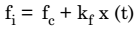where, x (t) is the modulating signal and the term kf x (t) represents the frequency deviation.

The constant kf will control the deviation. For small values of kf, the frequency deviation is small and the spectrum of FM signal has a narrow band. Hence, it is called as the narrow band FM.

Let us consider the expression for FM wave as under: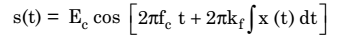Expressing it in terms of ω, we have: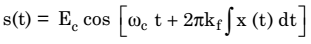We can represent this in the exponential manner as under: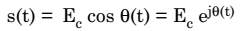This has been written by considering only the real part of Ec ejθ(t)

Therefore,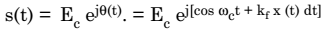Let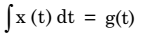Thus,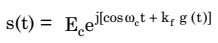If kf g (t) < < 1 for all values (which is the case for narrow band FM), then, the expression for FM will be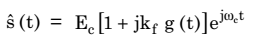Also,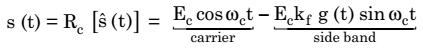This is the expression for narrowband FM.